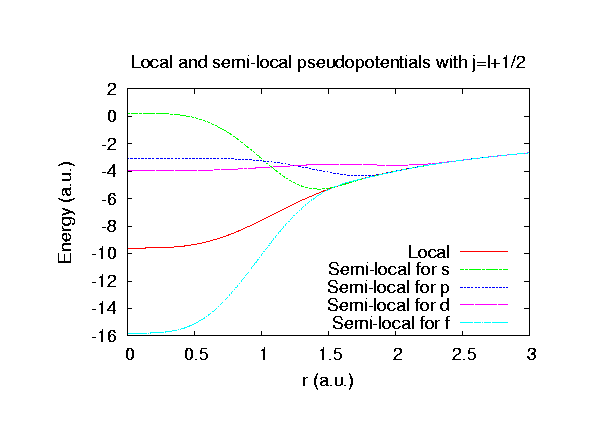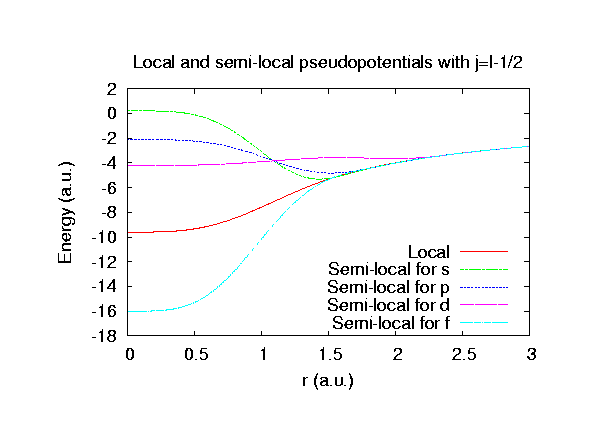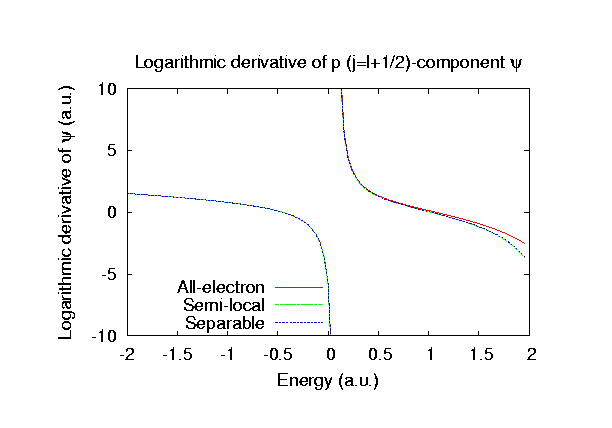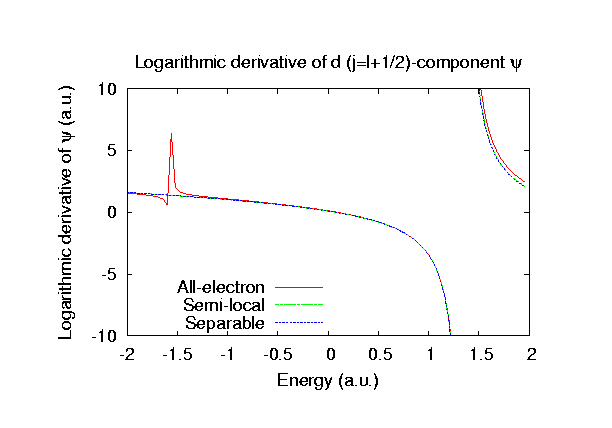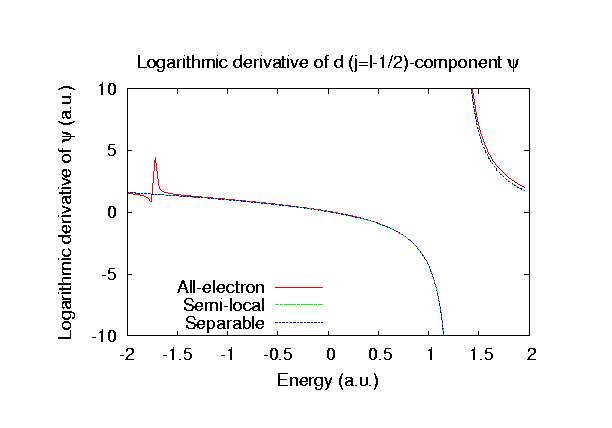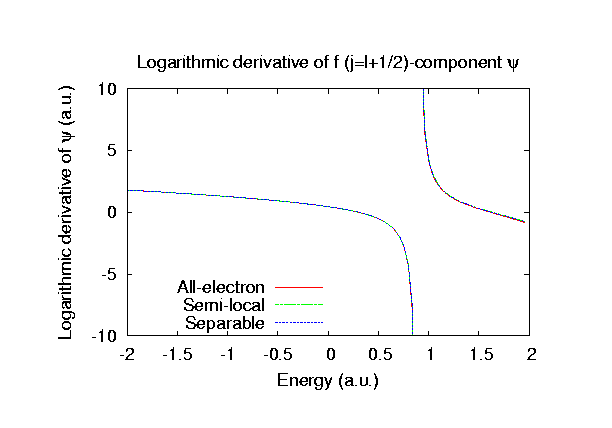### Fully relativistic pseudopotentials

Fully relativistic pseudopotentials generated by the MBK (PRB 47, 6728 (1993)) scheme within LDA (CA13) and GGA (PBE13) which contain a partial core correction and fully relativistic effects including spin-orbit coupling.

### Pseudo-atomic orbitals

The number below the symbol means a cutoff radius (a.u.) of the confinement potential. These file includes fifteen radial parts for each angular momentum quantum number l (=0,1,2,3). The basis functions were generated by ADPACK, and never optimized by OpenMX. Since Rn_CA13.vps and Rn_PBE13.vps include the 6s and 6p state (8 electrons) as the valence state, the minimal basis set is Rn*.*-s1p1.

### Benchmark calculations by the PBE13 pseudopotential

(1) Calculations of the band dispersion in the fcc structure, where the non-spin polarized collinear calculation with the lattice constant of 4.5 Ang. was performed using Rn_PBE13.vps and Rn11.0-s3p2d2f1, and the origin of the energy is taken to be the top of the valence band. The input file used for the OpenMX calculations can be found at Rnfcc-Band.dat. For comparison the result by the Wien2k code is also shown, where the calculation was performed by default setting in the Ver. 9.1 of Wien2k except for the use of RMT x KMAX of 12.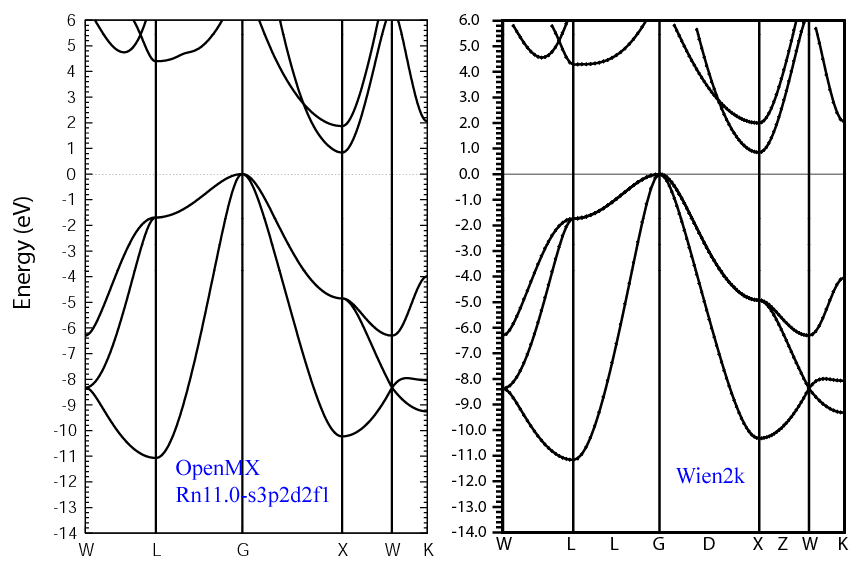### Supplementary information for the GGA (PBE13) pseudopotential